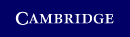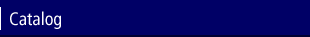Home > Catalog > PercolationSearch this bookDetails

• 112 b/w illus.
• Page extent: 334 pages
• Size: 228 x 152 mm
• Weight: 0.66 kg

Library of Congress

• Dewey number: 530.13
• Dewey version: 22
• LC Classification: QC174.85.P45 B65 2006
• Percolation (Statistical physics)

Library of Congress Record

Hardback

(ISBN-13: 9780521872324 | ISBN-10: 0521872324)

• Published September 2006

Manufactured on demand: supplied direct from the printer

\$99.99 (P)

Percolation theory was initiated some fifty years ago as a mathematical framework for the study of random physical processes such as flow through a disordered porous medium. It has proved to be a remarkably rich theory, with applications beyond natural phenomena to topics such as network modelling. The aims of this book, first published in 2006, are twofold. First to present classical results in a way that is accessible to non-specialists. Second, to describe results of Smirnov in conformal invariance, and outline the proof that the critical probability for random Voronoi percolation in the plane is 1/2. Throughout, the presentation is streamlined, with elegant and straightforward proofs requiring minimal background in probability and graph theory. Numerous examples illustrate the important concepts and enrich the arguments. All-in-all, it will be an essential purchase for mathematicians, physicists, electrical engineers and computer scientists working in this exciting area.

Contents

Preface; 1. Basic concepts; 2. Probabilistic tools; 3. Percolation on Z2 - the Harris-Kesten theorem; 4. Exponential decay and critical probabilities - theorems of Menshikov and Aizenman & Barsky; 5. Uniqueness of the infinite open cluster and critical probabilities; 6. Estimating critical probabilities; 7. Conformal invariance - Smirnov's theorem; 8. Continuum percolation; Bibliography; Index; List of notation.

Review

"... A useful addition for its focus on the recent advances in two-dimensional percolation..."
Rongfeng Sun, Mathematical Reviews